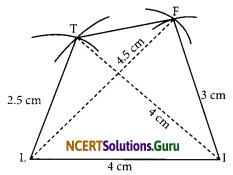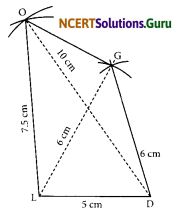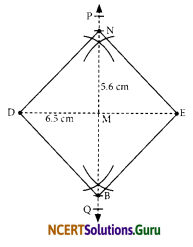# NCERT Solutions for Class 8 Maths Chapter 4 Practical Geometry Ex 4.2

These NCERT Solutions for Class 8 Maths Chapter 4 Practical Geometry Ex 4.2 Questions and Answers are prepared by our highly skilled subject experts.

## NCERT Solutions for Class 8 Maths Chapter 4 Practical Geometry Exercise 4.2

Question 1.
LI = 4 cm
IF = 3 cm
TL = 2.5 cm
LF = 4.5 cm
IT = 4 cmOL = 7.5 cm
GL = 6 cm
GD = 6 cm
LD = 5 cm
OD = 10 cm

(iii) Rhombus BEND
BN = 5.6 cm
DE = 6.5 cm
Solution:
(i)Steps of Construction:
I. Draw a line segment LI = 4 cm.
II. With centre at I and radius = 4 cm, draw an arc.
III. With centre at L and radius = 2.5 cm, draw another arc to intersect the previous arc at T.
IV. Join TI and TL.
V. With centre at L and radius = 4.5 cm, draw an arc on the side opposite to that of L.
VI. With centre at I and radius = 3 cm, draw another arc to intersect the previous arc at F.
VII. Join FL and FI.
Thus, LIFT is the required quadrilateral.(ii)Steps of construction:
I. Draw a line segment LD = 5 cm.
II. With centre at L and radius = 6 cm, draw an arc.
III. With centre at D and radius = 6 cm, draw another arc to intersect the previous arc at G.
IV. Join GL and GD.
V. With centre at D and radius = 10 cm, draw an arc.
VI. With centre at L at radius = 7.5 cm, draw another arc to intersect the previous arc at O.
VII. Join OL and OG.
Thus, GOLD is the required quadrilateral.(iii)Steps of construction:
I. Draw a line segment DE = 6.5 cm.
II. Draw perpendicular bisector of DE such that M is the mid-point of DE.
III. With centre M and radius = 2.8 cm, draw arcs to intersect the perpendicular bisector at B and N.
IV. Join ND, NE, BE, and BD.
Thus, BEND is the required rhombus.

error: Content is protected !!